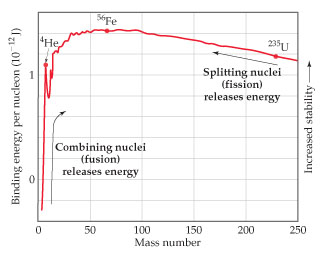# Problem: The atomic masses of hydrogen-2 (deuterium), helium-4, and lithium-6 are 2.014102 amu, 4.002602 amu, and 6.0151228 amu, respectively. For 4He, calculate the nuclear binding energy.

###### FREE Expert Solution

We are asked to calculate the nuclear binding energy for 4He.

To calculate for the nuclear binding energy:

Step 1: Determine the number of protons and neutrons

Step 2: Determine the total mass of protons and neutrons

Step 3: Calculate the mass defect (∆m)

Step 4: Determine nuclear binding energy per nucleon###### Problem Details

The atomic masses of hydrogen-2 (deuterium), helium-4, and lithium-6 are 2.014102 amu, 4.002602 amu, and 6.0151228 amu, respectively.For 4He, calculate the nuclear binding energy.